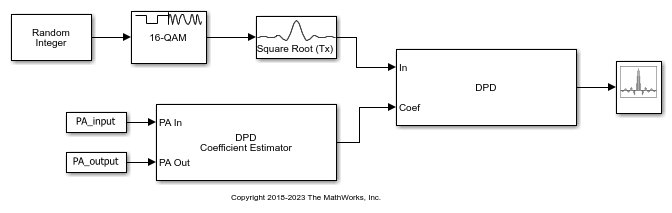# Predistort Power Amplifier Input Signal in Simulink

Apply digital predistortion (DPD) to a 16-QAM signal of random symbols. The DPD Coefficient Estimator block uses a captured signal containing power amplifier (PA) input and output signals to determine the predistortion coefficient matrix. Digital predistortion of the signal preconditions is to correct impairments that the PA introduces. This model does not include a block representing the PA.

The `File>Model Properties>Callbacks>PreLoadFcn` callback initializes model parameters, and loads workspace variables `PA_Input` and output `PA_Output` from the file `commpowamp_dpd_data.mat`. `PA_Input` and `PA_Output` variables are baseband-equivalent signals captured at the input and output of a PA. `PA_Input` and `PA_Output` are used by the DPD Coefficient Estimator block to estimate the memory-polynomial coefficients. The memory-polynomial coefficients are input to the DPD block to predistort the PA input signal.The input signal path of the model generates a random symbol stream, applies 16-QAM modulation, and applies raised-cosine transmit filtering to the modulated signal.

The input signal is digitally predistorted in the DPD block using the memory-polynomial coefficients generated in the DPD Coefficient Estimator block. Apply digital predistortion to the data. The DPD block returns the predistorted input signal for the PA that produced the distorted PA output signal, `PA_output`.